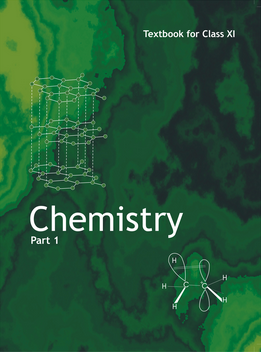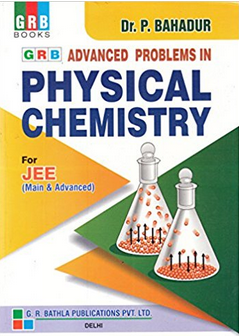# Chemical Thermodynamics   Share

## What is Chemical Thermodynamics

Chemical reactions, such as those that occur when you light a match, involve changes in energy as well as matter. Societies at all levels of development could not function without the energy released by chemical reactions. Almost 85% of energy consumption comes from the combustion of petroleum products, coal, wood, and garbage..We use this energy to produce electricity; to transport food, raw materials, manufactured goods; for industrial production; and to heat and power our homes. While these combustion reactions help us meet our essential energy needs.Chemical Energy stored by molecules can be released as heat during chemical reactions when a fuel like methane, cooking gas or coal burns in air. The chemical energy may also be used to do mechanical work when a fuel burns in an engine or to provide electrical energy through a galvanic cell-like dry cell. Thus various forms of energy are interrelated and can be transformed from one form into another. The study of these energy transformations forms the subject matter of thermodynamics. Thermodynamics is not concerned about how and at what rate these energy transformations are carried out but is based on initial and final states of a system undergoing the change.

Thermodynamics and its laws are immensely used in our daily life and it is very interesting to understand these processes. Some of them are mentioned below:

• The ice cubes in a drink absorb heat from it and make it cold and itself melt with time gradually. This flow of heat occurs according to the first and second law of thermodynamics.• The air conditioners in our homes also work on the principles of thermodynamics. It absorbs heat from the room and makes it cool. It throws this heat to the outside atmosphere, thus the backside of air conditioners are always warm.• The combustion of gasoline provides the energy to the car engines, due to which we are able to travel to different places.## Notes for Chemical Thermodynamics

In this section, you will study about the important topics of the chapter, overview, formulae and some important tips and guidelines for the preparation of the chapter at the best.

## Important Topics- Chemical Thermodynamics

• Basic Terminology
• Internal Energy
• First Law of Thermodynamics
• Work
• Heat Capacity
• Enthalpies for different types of reactions
• Spontaneity
• Gibbs Energy

## Overview of the Chapter

A system is something in which observations related to heat and work are done. Other than the system, everything else existing is known as the surroundings. In thermodynamics, first you need to understand various kinds of important terms as follows:

• Open System: It is the system, where the exchange of matter and energy takes place between the system and surroundings.
• Closed System: It is the system, in which exchange of matter does not take place but the exchange of energy is possible between the system and surroundings.
• Isolated System: In this system, no exchange of matter and energy is possible between the system and the surroundings.
• Adiabatic process: It is the process, in which transfer of energy does not take between the system and the surroundings.
• Intensive properties: These are those properties of the system which do not depend upon the quantity or size of the system. Common examples include temperature, density, pressure, etc.
• Extensive properties: These are those properties of the system which depends upon the quantity or size of the system. Some examples include mass, volume, internal energy, etc.
• Internal Energy: It is the total energy of the system. Energy can be of any type like mechanical, electrical, etc thus the sum of all these energies is known as the internal energy.

First Law of Thermodynamics

This law simply states that "energy of an isolated system is constant". In other words, energy can neither be created nor be destroyed. Mathematically, it can be expressed as follows:

Work

In thermodynamics, work is defined as the force that we applied on the system to change its volume form Vi to Vf.Mathematically, work can be described as follows:

Now, if the pressure is not constant but is changing infinitesimally, then work is given as follows,

Heat Capacity

When heat is applied to any system, the temperature of the system rises. But if the same amount of heat energy is given to different systems, then these systems rise with different temperatures. This variation of temperatures in different systems is only because of coefficient or heat capacity. This heat capacity is denoted by C. Thus, mathematically, heat capacity can be expressed as follows:

• Relationship between Cp and Cfor an ideal gas:
At constant volume, the equation of heat can be written as:

And at constant pressure, the equation of heat can be written as :

After the derivation, we have found the relation between Cp and Cv as follows:

Enthalpies for different types of reactions

There are various kinds of enthalpies for various kinds of reactions. These are mentioned below:

• Standard enthalpy of combustion(): When one mole of a substance is heated and form the products, then the enthalpy change during this reaction is known as standard enthalpy of combustion.
• Enthalpy of atomization(): The enthalpy change when one mole of bonds are broken to obtain atoms in the gas phase is known as enthalpy of atomization.
• Enthalpy of solution(): When one mole of a substance is dissolved in a solvent then the enthalpy change occurs, in this case, is known as enthalpy of solution.
• Lattice enthalpy: This is the enthalpy change when one mole of an ionic compound dissociates into its ions in the gaseous state.

Spontaneity: In simple words, a spontaneous process is a process which occurs on its own. This process might be too fast or too slow, but it does not require any external agency to occur. It is always irreversible and can only be reversed by external things. For example, the reaction of hydrogen and oxygen is very slow at normal conditions but still, it is a spontaneous reaction as it is occurring on its own.

Gibbs energy and spontaneity

Gibbs energy is an extensive property and a state function. It gives us information about the spontaneity of any reaction. It collective and takes into account both the enthalpy and the entropy. Mathematically, it can be formulated as follows:

For determining the spontaneity of any process, two cases arise for Gibbs energy as follows:

• If , the process is spontaneous.
• If , the process is non-spontaneous.

## How to prepare for Chemical Thermodynamics?

• This chapter is a part of Physical chemistry. This chapter is one of the most important chapters of the complete chemistry syllabus. Its concepts, laws, numerical and graphs all are important both for the basic foundation of chemistry and for scoring good marks in the examination.

• Before reading this chapter, first, you must have the basic knowledge of the mole concept.

• You must observe carefully about the enthalpies, heat capacities, entropy, etc.

• Rest this chapter is very simple, just be regular and be consistent in your numerical practice.

## Prescribed Books for the Chemical Thermodynamics

First, you must finish the class XI NCERT textbook and solve each and every example and unsolved question given in it. Then for advanced level preparation like JEE and NEET, you must follow R.C. Mukherjee and O.P. Tandon. You must definitely solve the previous year papers. Meanwhile, in the preparation, you must continuously write the mock tests for the depth of knowledge. Our platform will help you to provide with the variety of questions for deeper knowledge with the help of videos, articles and mock tests.

## Chemistry Chapter- wise Notes for Engineering and Medical Exams

 Chapters No. Chapters Name Chapter 1 Some basic concepts in chemistry Chapter 2 States of matter Chapter 3 Atomic Structure Chapter 4 Solutions Chapter 6 Equilibrium Chapter 7 Redox Reaction and Electrochemistry Chapter 8 Chemical kinetics Chapter 9 Surface Chemistry Chapter 10 General Principle and processes of Isolation of metals Chapter 11 Classification of Elements and Periodic table Chapter 12 Hydrogen Chapter 13 p- block Elements Chapter 14 s-block Elements (Alkali and Alkaline Earth Metals) Chapter 15 Chemical Bonding and Molecular Structure Chapter 16 d- and f- BLOCK ELEMENTS Chapter 17 Coordination Compounds Chapter  18 Environmental Chemistry Chapter 19 Purification and Characterisation of Organic Compounds Chapter 20 Some Basic Principles of Organic Chemistry Chapter 21 Hydrocarbons Chapter 22 Organic Compounds containing Halogens Chapter 23 Organic Compounds containing Oxygen Chapter 24 Organic Compounds Containing Nitrogen Chapter 25 Polymers Chapter 26 Biomolecules Chapter 27 Chemistry in Everyday Life Chapter 28 Principles Related to Practical Chemistry

### Topics from Chemical Thermodynamics

• Fundamentals of thermodynamics: System and surroundings ( JEE Main, MET ) (20 concepts)
• First law of thermodynamics - Concept of work ( JEE Main, MET ) (34 concepts)
• First law of thermodynamics-internal energy and enthalpy ( JEE Main, MET ) (4 concepts)
• heat capacity and specific heat ( JEE Main, MET ) (14 concepts)
• Second law of thermodynamics ( JEE Main, MET ) (20 concepts)
• Gibbs energy change for spontaneous and non-spontaneous process ( JEE Main, MET ) (8 concepts)
• Criteria for equilibrium and spontaneity ( JEE Main, MET ) (2 concepts)
• combustion ( JEE Main, MET ) (2 concepts)
• measurement of Delta U and Delta H ( JEE Main, MET ) (6 concepts)
• Hydration, ionization and solution. ( JEE Main, MET ) (2 concepts)
• enthalpy of : bond dissociation ( JEE Main, MET ) (2 concepts)
• phase transition ( JEE Main, MET ) (2 concepts)
• atomization ( JEE Main, MET ) (2 concepts)
• Sublimation ( JEE Main, MET ) (2 concepts)
• solution and dilution ( JEE Main, MET ) (2 concepts)
• Hess's law of constant heat summation ( JEE Main, MET ) (2 concepts)
• formation ( JEE Main, MET ) (2 concepts)
• INTRODUCTION TO THERMODYNAMICS ( JEE Main, MET ) (2 concepts)
• THERMODYNAMIC PROCESS ( JEE Main, MET ) (2 concepts)
• THERMODYNAMIC EQUILIBRIUM ( JEE Main, MET ) (1 concepts)
• LAWS OF THERMODYNAMICS ( JEE Main, MET ) (7 concepts)
• HEAT CAPACITY AND MOLAR HEAT CAPACITY ( JEE Main, MET ) (2 concepts)
• TERMS USED FOR HEAT OF REACTION ( JEE Main, MET ) (11 concepts)
• MEASUREMENT OF ENTHALPY OF REACTION ( JEE Main, MET ) (1 concepts)
• ENTROPY ( JEE Main, MET ) (2 concepts)
• SPONTANEOUS PROCESS ( JEE Main, MET ) (2 concepts)
• SECOND LAW OF THERMODYNAMICS ( JEE Main, MET ) (1 concepts)
• SPONTANEITY ( JEE Main, MET ) (3 concepts)
• THIRD LAW OF THERMODYNAMICS ( JEE Main, MET ) (1 concepts)
• SMART TIPS: CHEMICAL THERMODYNAMICS ( JEE Main, MET ) (1 concepts)

### Important Books for Chemical Thermodynamics

••Exams
Articles
Questions